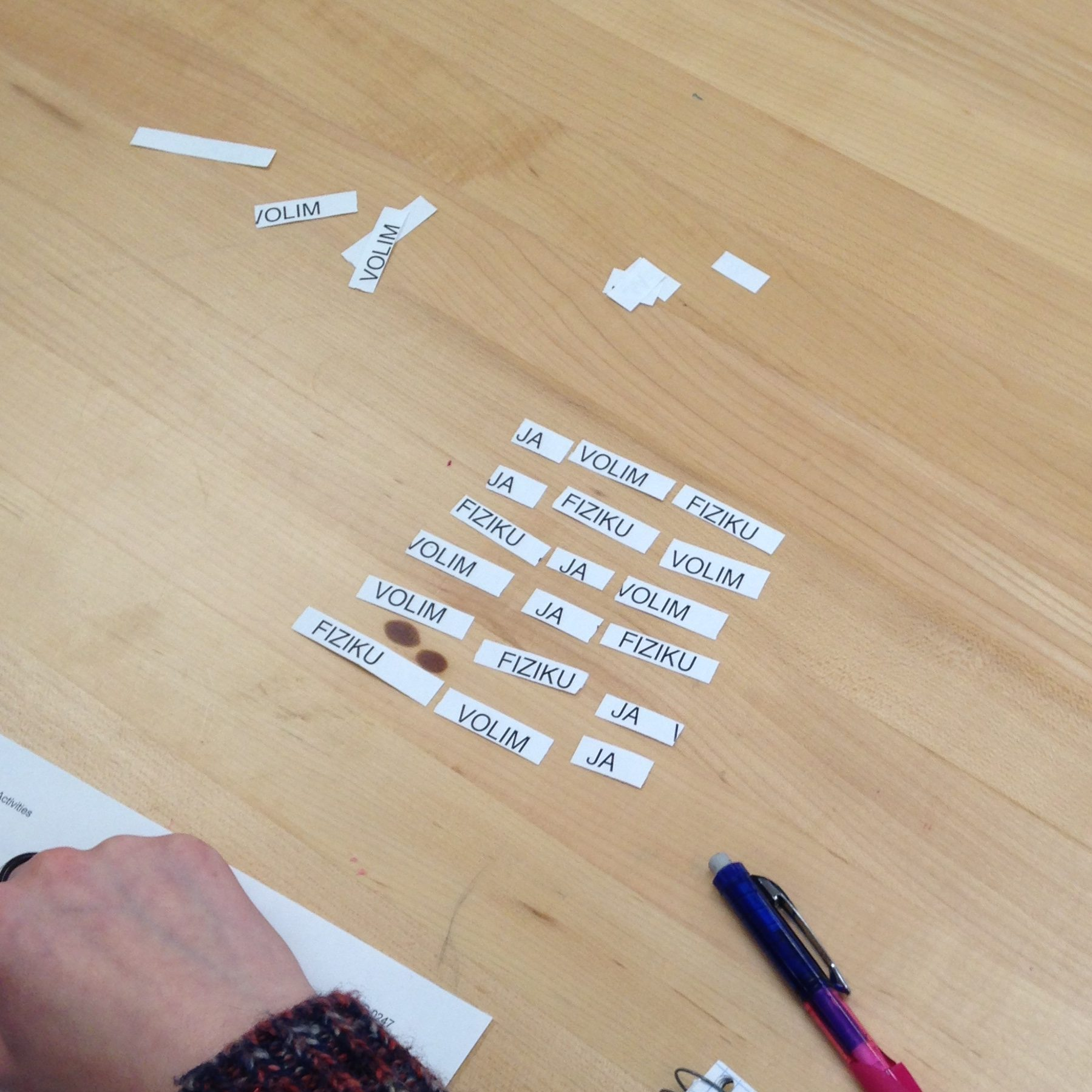On Day 11, we began with all the grammatically correct ways to say “I love physics” in Bosnian/Croatian/Serbian language. That happens to be a total of 3!=6 ways, each a permutation of “JA VOLIM FIZIKU,” which is pronounced roughly as |ya volim feezikoo|. I couldn’t resist the temptation to bring in my native tongue(s) into the lecture; the students mostly rolled with it. 🙂

• The class  started with students constructing the sentences by arranging thee pieces of paper with individual words in all possible orders.All the ways “I love Physics.”

• Once we figured out all the possible combinations of the three words, we gave a symbol to each permutation taking the original sentence to a different one, as well as the identity.Introducing notation for permutations.

• After we clarified what it means to apply permutations successively, students wrote out the multiplication table for the permutations. This was particularly interesting to me because each student seemed to have a different method of tracking the changes after each permutation. Some moved the pieces of paper, some sketched the process by writing the string of words after each permutation, and one kept track of the permutations by creating a “dictionary ” pictured below which he used to track the changes. A subtle question arose of whether the first subsequent permutation was to be applied to the specific words used in the definition of the permutation, or on whichever words were in the original locations of the words from the definition.Working on the multiplication table for permutations.

• We inspected the multiplication table to find that these permutations form a group, which led to a formal introduction of the symmetric group S3, and the symmetric groups of permutations in general.
• We concluded the class by drawing parallels to D3, which is isomorphic to S3.